Latest Banking jobs   »

# Quantitative Aptitude Quiz For SIDBI Grade A Prelims 2023- 11th January

Q1. The difference of length & breadth of a rectangle is equal to 24cm and ratio of area of rectangle to area of square is 5 : 9. If the perimeter of rectangle is 72 cm. find the volume of cone given that height of cone is equal to breadth of rectangle and radius is equal to side of square (Length of rectangle is greater than its breadth).
(a) 624π cm³
(b) 726π cm³
(c) 824 π cm³
(d) 648π cm³
(e) None of these

Q2. The ratio of age of Amit and his father 5 years ago is 2 : 5. If age of his mother is 20% more than the present age of his father. Then find difference of age of his father and mother after 10 years given that age of Amit 3 year hence will be 32 years?
(a) 11 years
(b) 13 years
(c) 8 years
(d) 17 years
(e) 15 years

Q3. The number of girls in the college was 50 less than the number of boys in that college. Subsequently, a few number of girls joined that college and the ratio of boys and girls become 2 : 5. Find the minimum number of girls who joined college recently (Number of boys and girls are positive integer).
(a) 116
(b) 120
(c) 124
(d) 128
(e) 136

Q4. Average weight of group of some children increases by 1 when 2 children join them. If instead of these 2 children another 2 children join the group, then average decreased by 1 but total weight becomes more than initial weight. If difference between the sums of weight of 2 children of previous one to later one is 14 then find the initial number of children in group.
(a) 7
(b) 5
(c) 3
(d) 6
(e) None of these

Q5. A right-angled triangle, having area 42 cm², is rotated along its base, as an axis, formed a cone. Curved surfaced area of such cone is increased by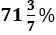when it rotates along its height. What is the volume of cone, that formed when triangle rotate along its Base?
(a) 1056 cm³
(b) 1024 cm³
(c) 616 cm³
(d) 918 cm³
(e) None of these

Q6. The ratio of average of two series of five numbers each is 2:3. All five terms of the former series are consecutive multiples of 4 while all five terms of the latter series are consecutive multiples of 6. If sum of their first term is 20, then find the difference between greatest term of series divisible by 6 and second greatest term of series divisible by 4?
(a) 16
(b) 12
(c) 10
(d) 14
(e) 8

Q7. A sum of Rs ‘x’ becomes Rs (x + 528) in two years at the rate of 20% p.a compounded annually. if Veer invested Rs 2.5x at 15% p.a. on SI for three years and Ayush invest Rs 1.5x at 10% on CI for two years, then find the ratio of interest got by Ayush to that of Veer?
(a) 4 : 25
(b) 6 : 25
(c) 8 : 25
(d) 7 : 25
(e) None of these

Q8. Distance between point A and Delhi Airport is 3600 km. If a pilot gets late by 50 minutes, then to reach Delhi Airport from point A at scheduled time, he has to increase the speed of plane by 20%. Then find distance traveled by plane in 3 hr 45 minutes, if it travels with increased speed?
(a) 2840 km
(b) 3240 km
(c) 3280 km
(d) 3640 km
(e) 3840 km

Q9. A person employs 10 men to finish a task in 90 days on a condition that no one will work on rainy days. On 51st day, after rain of 5 days, person finds that only 40% of the total work has been completed and thus employs 5 more men and, in this way, total work gets completed in 86 days including 11 rainy days. Find the percentage increase in the efficiency of a man.
(a) 50%
(b) 40%
(c) 60%
(d) 75%
(e) 80%

Q10. Difference of speed of boat in downstream and upstream is 7km/hr and the time taken by the boat to travel 45 km in downstream is 80 minutes more than the time taken to travel 13 km in upstream. Due to cyclone, speed of current becomes twice and thus it can cover D km in upstream in time which is equal to new speed of current. Find D? (note∶speed of boat in still water is multiple of 5)
(a) 30
(b) 24
(c) 18
(d) 21
(e) 27

Q11. A and B are two alloys which were made by mixing iron and copper in the ratio of 3 : 5 and 5 : 9 respectively. If 60 grams of alloy A and X grams of alloy B are melted and mixed to form another alloy C, what is the value of X if the ratio of iron and copper in the new alloy is 35 : 61 ?
(a) 70 gms
(b) 56 gms
(c) 98 gms
(d) 84 gms
(e) 112 gms

Q12. In a class there are two sections i.e. A and B. 25% of the students in section A and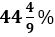of the students in section B are girls. If two students are chosen at random one each from section A and section B as class representative then find the probability that there will be exactly one boy out of chosen two students?
(a) 17/36
(b) 13/36
(c) 1/2
(d) 7/18
(e) 4/9

Q13. Ravi and Sneha got married 10 years ago and at that time ratio of their ages was 5:4. Ratio of present age of Ravi and Sneha is 7:6. After marriage they had seven children including a triplet and a twin. The ratio of present age of triplet, twin, sixth and the seventh child is 4:3:2:1. Find the largest possible value of the present total age of the family.
(a) 121
(b) 107
(c) 101
(d) 93
(e) None of the above

Q14. Shivam invested Rs 3 lac in a scheme which is providing interest rate of ‘r’% per annum at CI and this scheme doubles the sum invested in 72/r years which is two times of rate of interest provided by the scheme. Find the total amount received by Shivam at the end of 48 years (in lac)?
(a) 45
(b) 50
(c) 64
(d) 32
(e) 48

Q15. Marked price of two articles A and B is in the ratio of 3:4. Shopkeeper sold article A and article B at the discount of x % and (x+8) % respectively. Shopkeeper made a profit of 20% on selling article B and found that its cost price is equal to selling price of article A. Find the marked of article B when article A is sold for Rs 972 at two successive discounts of x/2% and 2x%?
(a) Rs 1800
(b) Rs 2000
(c) None of the above
(d) Rs 1600
(e) Rs 2800

Solutions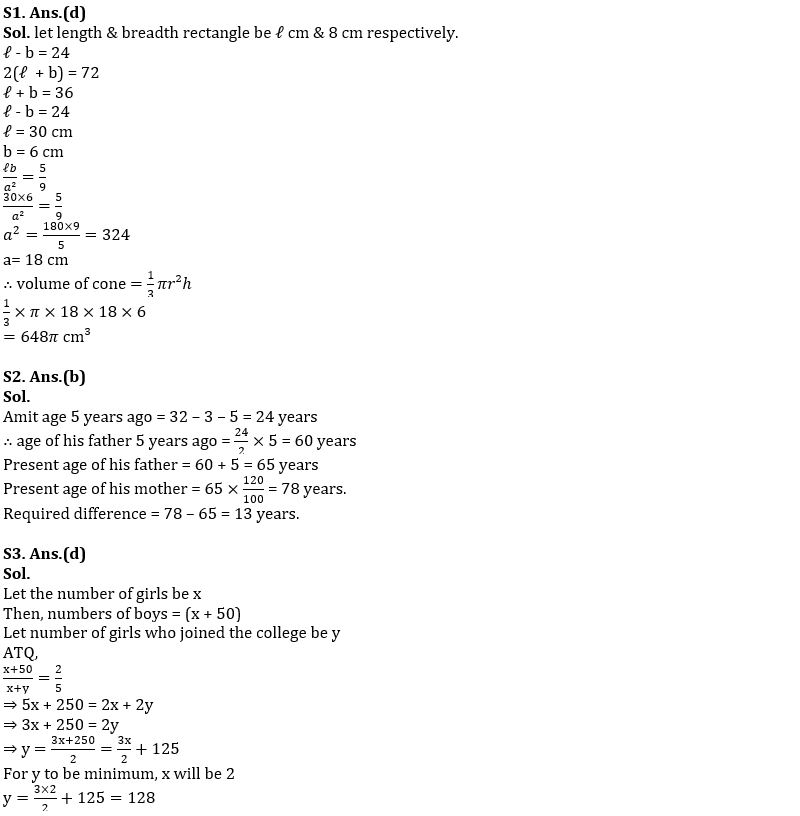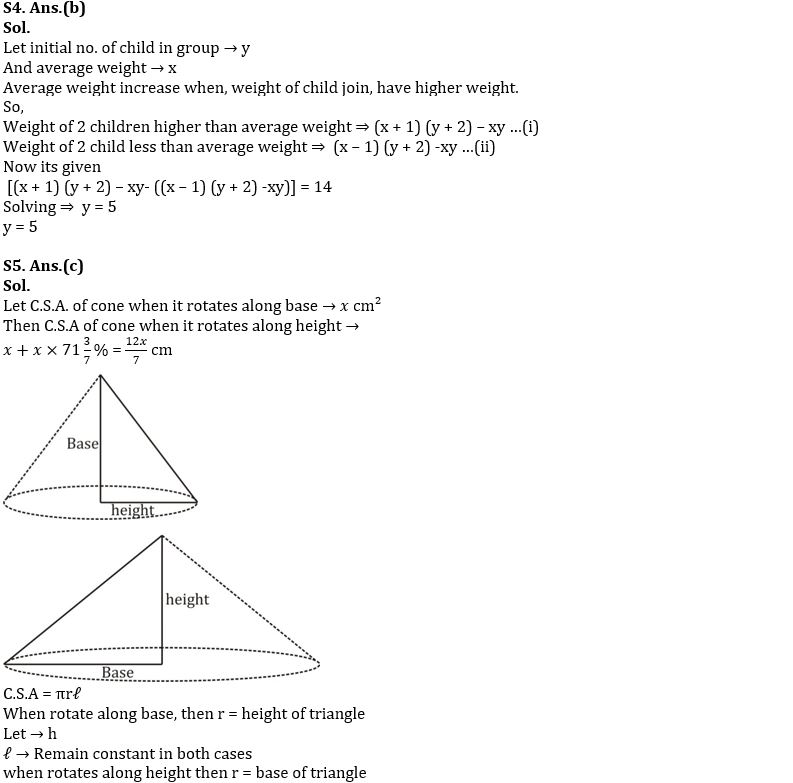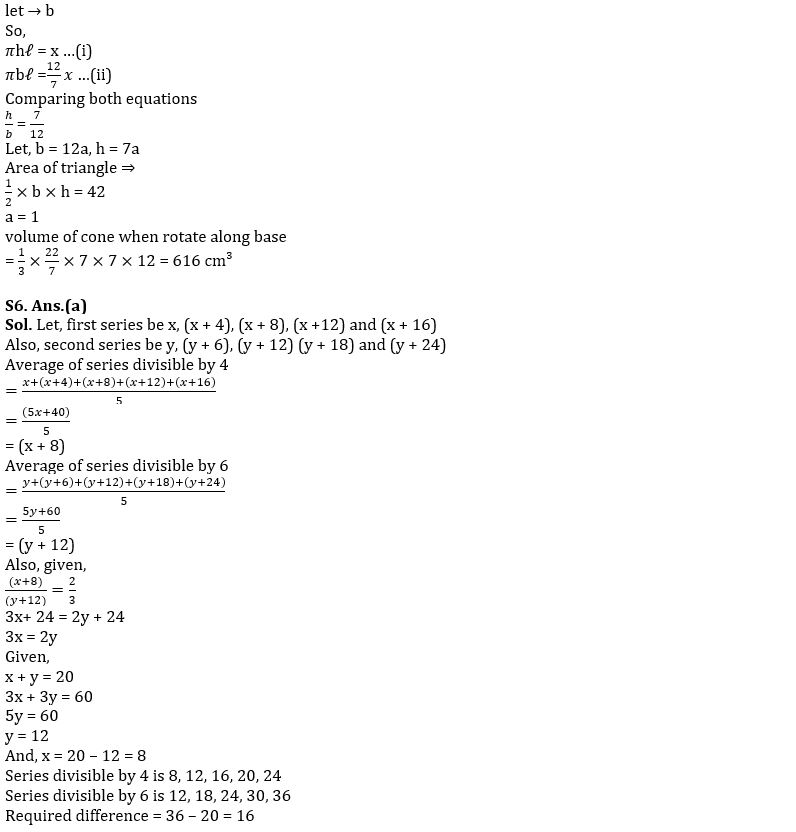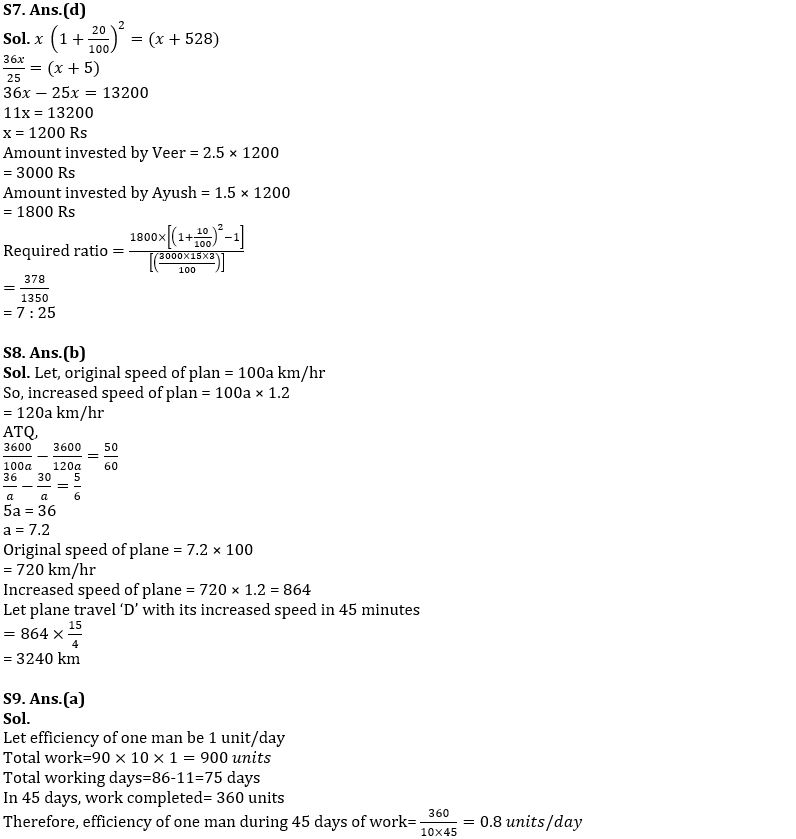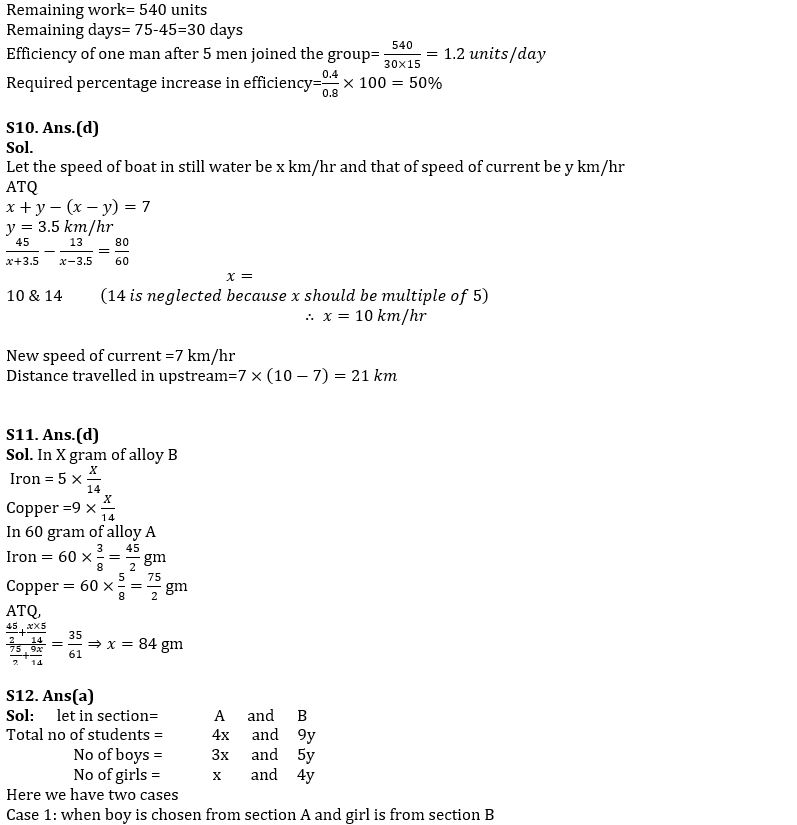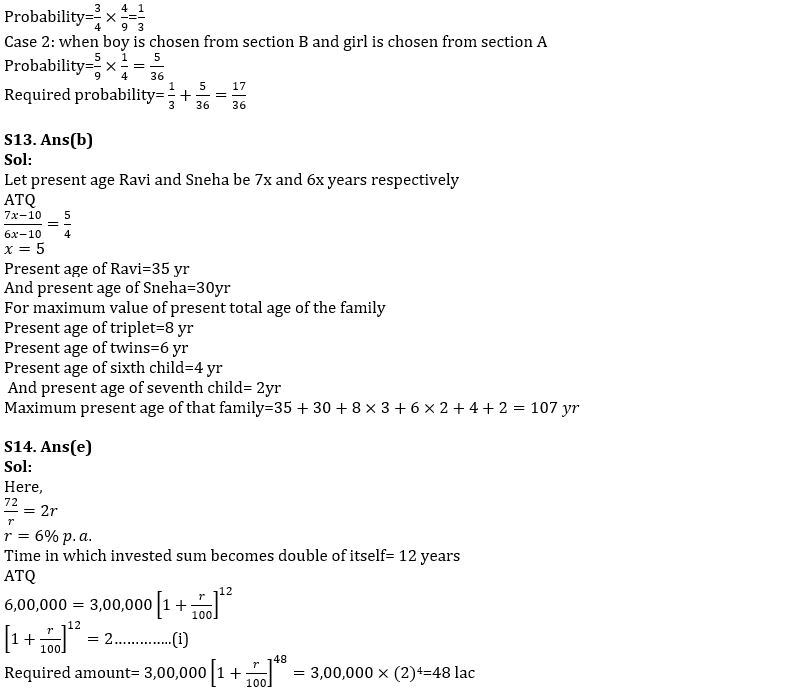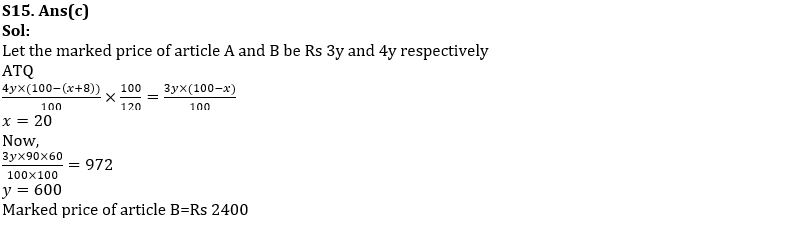## FAQs

### When will the SIDBI Grade A Exam 2023 be held?

The SIDBI Grade A Exam 2023 will be held on 28th January 2023.

#### Congratulations!